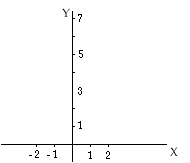I need help with grahing linear functions.If you could e-mail me back the basics and how tos I would be much appriciative.

Thank you
-Chelsea Ralya gr.7

Hi Chelsea,

I am going to illustrate with an example. Some time ago we were asked to make a chart of values for the linear function y = -2x + 3. We constructed the table

xy
03
11
-27

To plot the linear function y = -2x + 3 first draw the coordinate axes.Next plot the points from tha table above

Join the points with a

Add some points yourself. Increase the table to add points with the X-coordinate 2 and -1 and see if the points you get lie on the line.

I hope this helps,
Penny
Go to Math Central